• Octave 中的矩阵元素Octave中的矩阵和Matlab中的用法基本相同，但是为了明确一下，写出来具体的例子方便大家理解生成一个一般的矩阵：&gt;&gt; A=[1,2,3;4,5,6;7,8,9;] %逗号用来分隔每一行的元素，分号是...
Octave 中的矩阵元素
Octave中的矩阵和Matlab中的用法基本相同，但是为了明确一下，写出来具体的例子方便大家理解
生成一个一般的矩阵：

>> A=[1,2,3;4,5,6;7,8,9;] %逗号用来分隔每一行的元素，分号是换行
A =
1   2   3
4   5   6
7   8   9
对某个元素获取：
>> a12=A(1,2)
a12 =  2
>> a21=A(2,1)
a21 =  4
对某一行的获取：
>> A1i=A(1,:)
A1i =

1   2   3
对1、3两行获取：
>> A([1,3],:)
ans =

1   2   3
7   8   9
对某一列的获取：
>> Aj1=A(:,1) %提取第一列
Aj1 =
1
4
7
对1、3两列的获取：
>> A(:,[1,3])
ans =

1   3
4   6
7   9
元素遍历顺序说明：
>> A1=A(1)
A1 =  1
>> A2=A(2)
A2 =  4
>> A3=A(3)
A3 =  7
>> A4=A(4)
A4 =  2
可以看出编号大小的排列是先遍历列再遍历行
展开全文• 这个属于补充，从原来的矩阵进行变换生成新的矩阵&gt;&gt; A=[1,2,3;4,5,6;7,8,9;] A = 1 2 3 4 5 6 7 8 9从A当中把第一列和第三列抽出形成新的矩阵B&gt;&gt; B=A(:,[1,3]) B = 1 3 4 6 7 9...
这个属于补充，从原来的矩阵进行变换生成新的矩阵
>> A=[1,2,3;4,5,6;7,8,9;]
A =

1   2   3
4   5   6
7   8   9
从A当中把第一列和第三列抽出形成新的矩阵B
>> B=A(:,[1,3])
B =

1   3
4   6
7   9
把A当中的第1行、第2行，第2列、第3列抽出形成新的矩阵C
>> C=A([1,2],[2,3])
C =

2   3
5   6
现在把B C拼接
>> [B C]
error: horizontal dimensions mismatch (3x2 vs 2x2)
>> [B;C]
ans =

1   3
4   6
7   9
2   3
5   6

>> [B,C]
error: horizontal dimensions mismatch (3x2 vs 2x2)可以看到拼接需要匹配。


展开全文• ## octave矩阵运算

千次阅读 2018-04-05 16:55:29
A.*B A点乘B就是对应元素直接相乘 A.^2直接每个元素平方 log(e)=1 exp(1)=e abs(-1)=1 1*B做除法必须1./B [value,index]=max(a)最大的元素的位置，value是它的大小，index是他的位置 Max(A)会返回一个维度值个数的较...
A.*B A点乘B就是对应元素直接相乘
A.^2直接每个元素平方
log(e)=1
exp(1)=e
abs(-1)=1
1*B做除法必须1./B
[value,index]=max(a)最大的元素的位置，value是它的大小，index是他的位置
Max(A)会返回一个维度值个数的较大值
1 5 7
2 4 6会返回5 6 7，
A<3 return  if it's smaller than 3
[roll, colum]=find (A<3) returns the position when it's smaller than 3
magic(3) returns a magic square which sum of each row=sum of each collum 甚至是对角线。
sum(a);prod(a);floor(a);ceil(a);prod means *;floor means 0.4~~=0
max(rand(3),rand(3)) pick the bigger one in each count position,no max(rand(3),rand(3),rand(3));
I'm sneezing like in every 2 second,god help my nose.
max(A,[],1/2)行最大，列最大
flipud(eye(2))是上下对称一下
1 0      0 1
0 1=》 1 0
plot(x,y);%x=[0:0.1:1];
hold on;
xlable('size');
legend('name1','name2')
title('my pic')
print -dpng 'name';
figure(1);plot(t,y1);
subplot(1,2,1);分成横向一个，纵向两个的格子，用第一个作图
axis（[0.5 1 -1 1]）
clf;
imagesc(A)
iamgesc,colorbar,colormap gray

展开全文• 操作两个矩阵 合并两个矩阵： A.*B 会把A中的元素与B中的一一对应乘起来 1./N 对矩阵N里的所有元素都取倒数 log(N) 对矩阵N内的元素进行对数运算 exp(N) 对矩阵N内的元素进行以e为底元素为指数的幂运算 abs(N)...
操作两个矩阵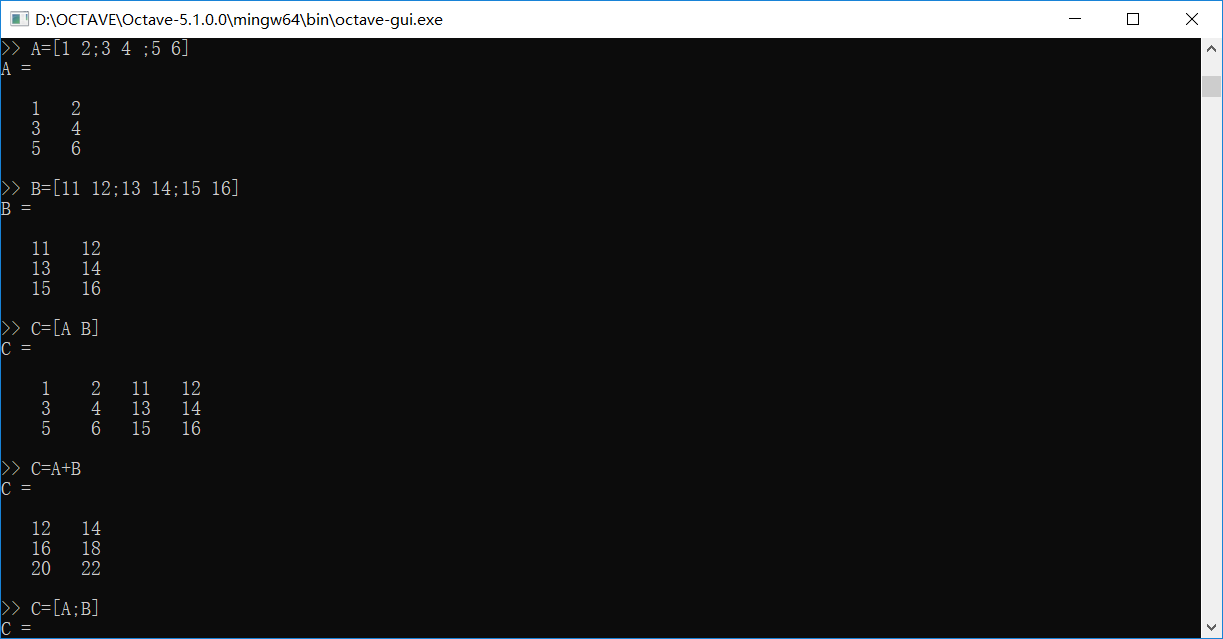合并两个矩阵：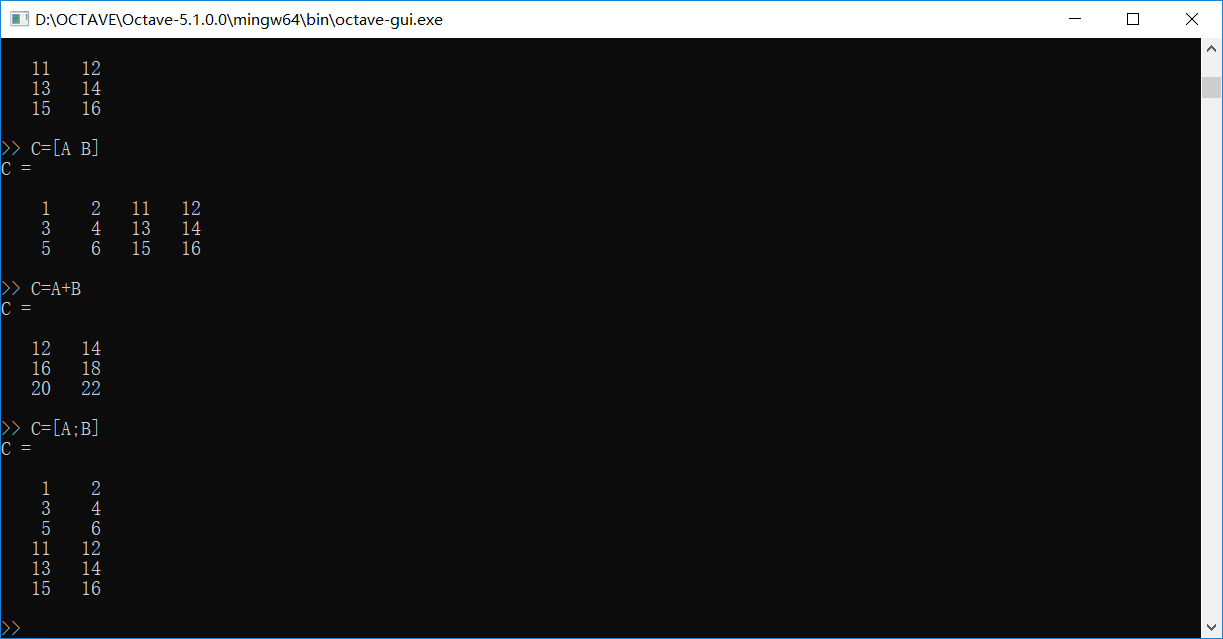A.*B 会把A中的元素与B中的一一对应乘起来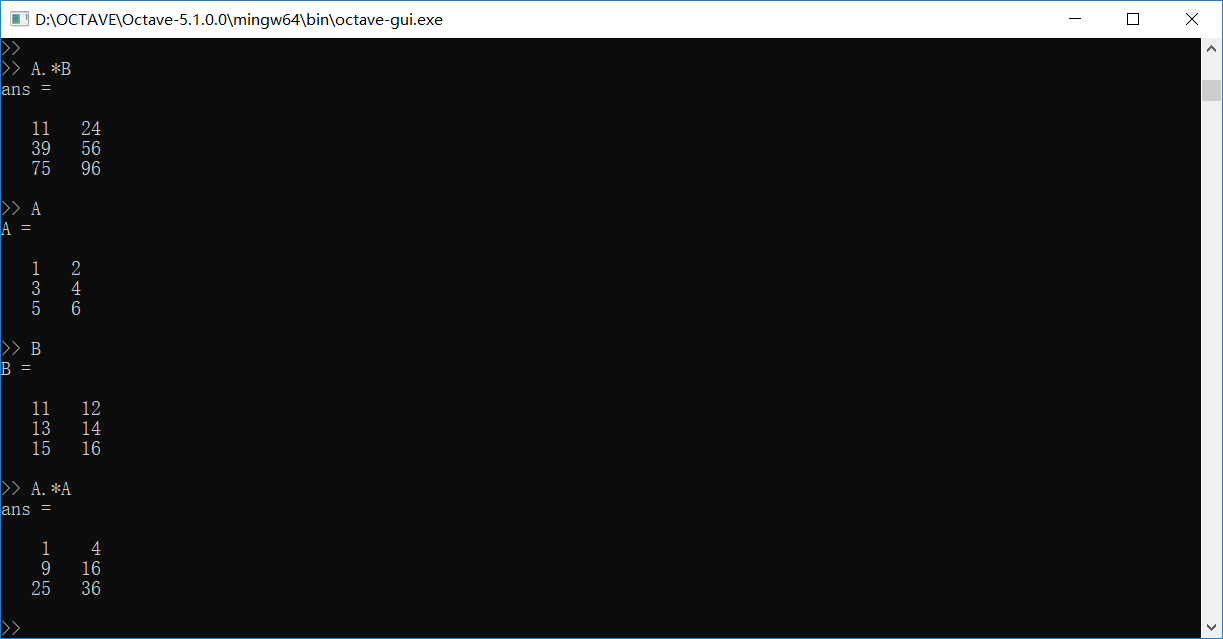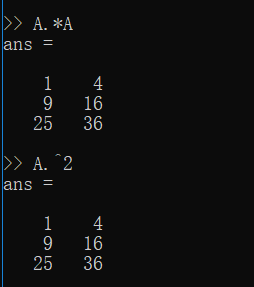1./N 对矩阵N里的所有元素都取倒数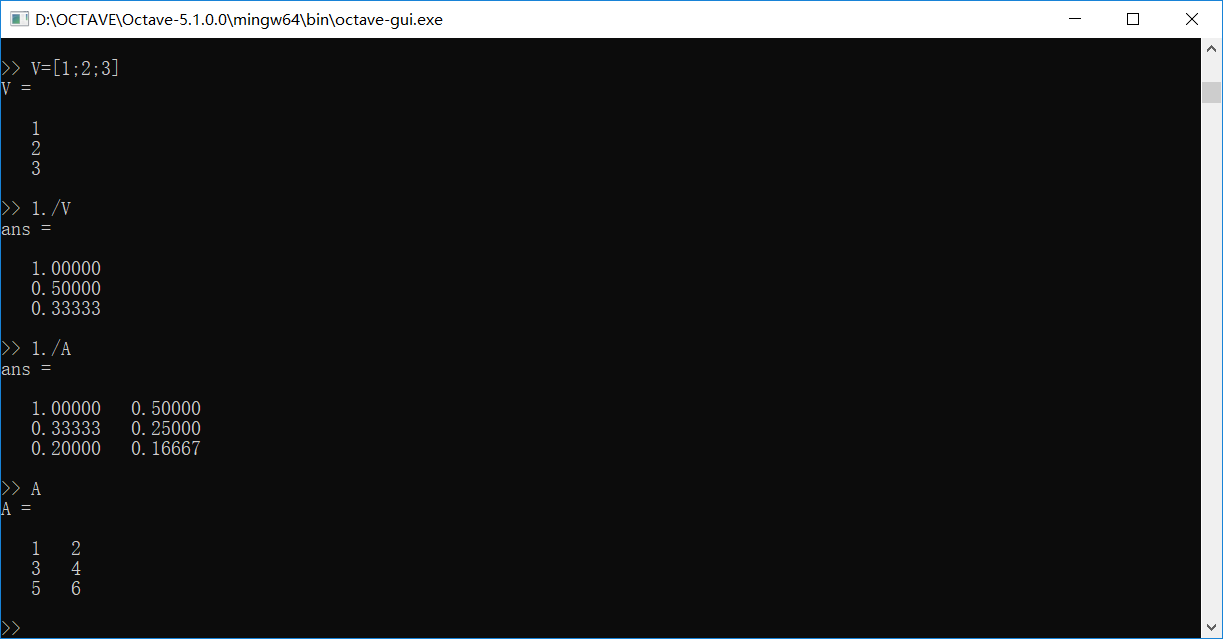magic(n) 返回名为幻方的n阶矩阵（任意行列以及对角线元素的值加起来都等于相同的值）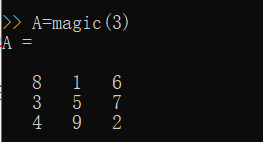log(N) 对矩阵N内的元素进行对数运算 exp(N) 对矩阵N内的元素进行以e为底元素为指数的幂运算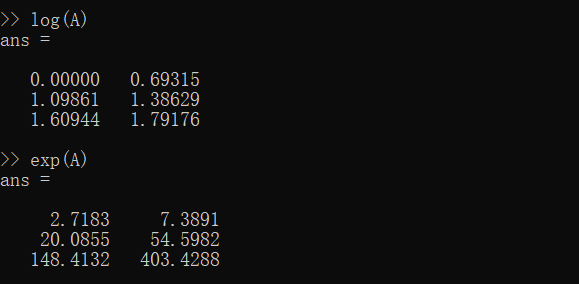abs(N) 矩阵内的元素全部去绝对值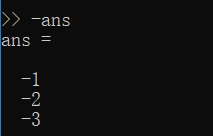骚操作加法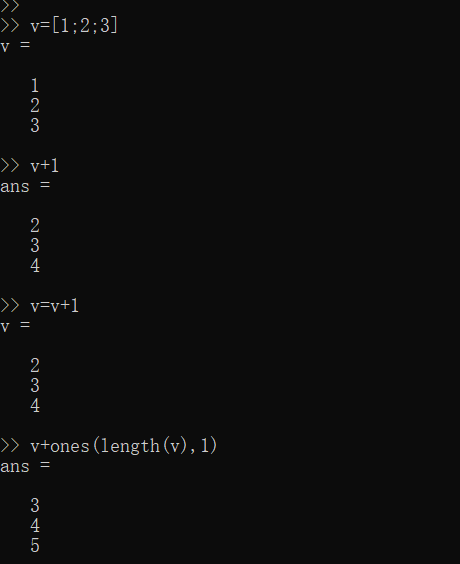求A的转置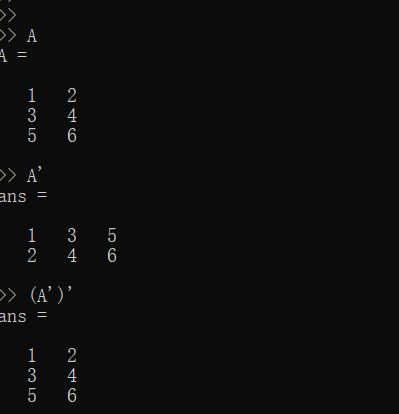取矩阵中元素的最大值 max（A） 下图中的ind是获得索引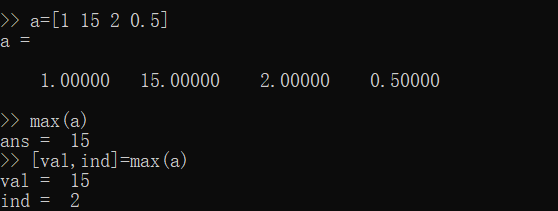当A为矩阵，默认返回每列的最大值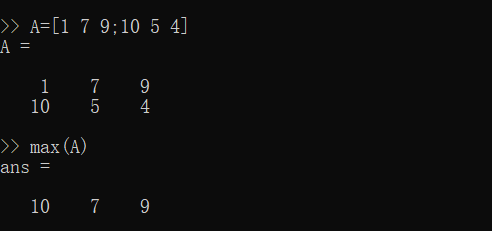1指定从列开始 2指定从行开始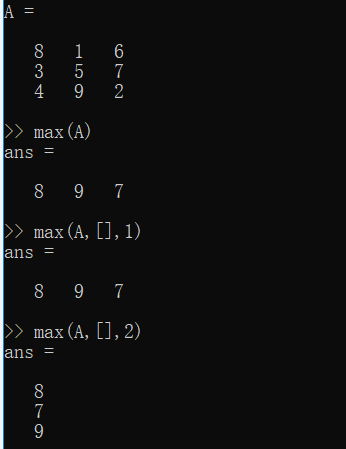max(A,B) 返回两个矩阵阵中对应元素的最大的那个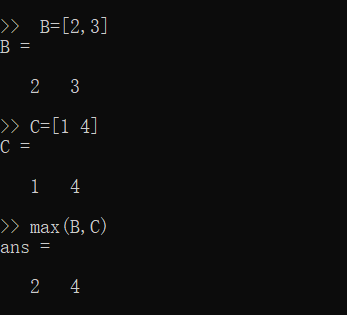矩阵比较返回1或0 find() 返回满足条件的下标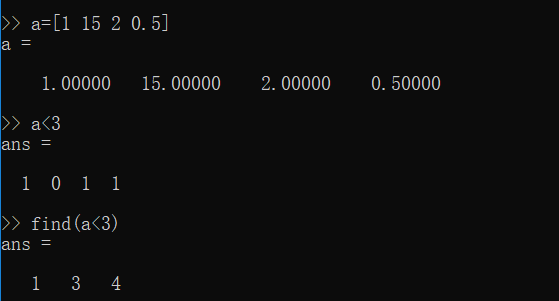find r代表行 c代表列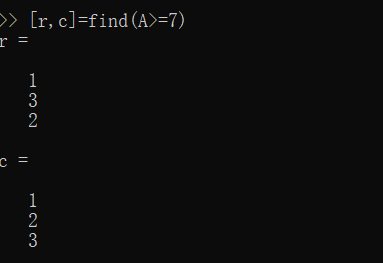求和函数sum() 求积函数prod() 去掉尾数floor() 四舍五入ceil()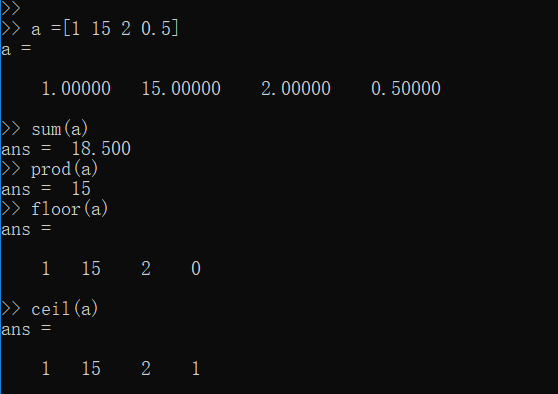求逆矩阵 pinv(A)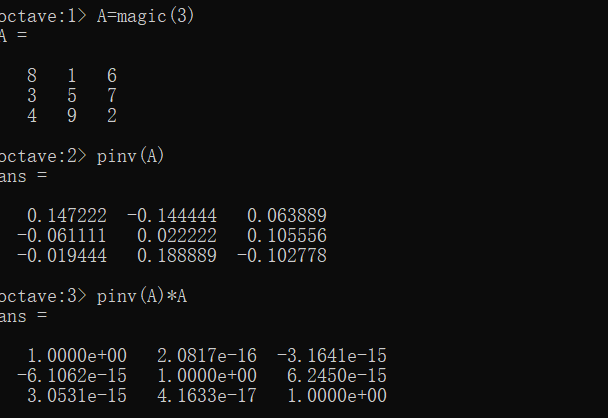展开全文• 33 22] error: vertical dimensions mismatch (1x1 vs 1x2) >> a([1 3],2) = [22 33 22] error: =: nonconformant arguments (op1 is 2x1, op2 is 1x3) %两个矩阵横向组合 >> a = [a [123; 3445;2346]] a = 1 22 ...
• 矩阵运算中的乘法，分为几种，下面从元素和矩阵两个角度进行举例说明。&gt;&gt; A=[1,2,3;4,5,6;7,8,9;] A = 1 2 3 4 5 6 7 8 9分别对矩阵本身做平方运算和每个元素做平方运算&gt;&gt; A.*2 ans...
• ## octave矩阵声明操作

千次阅读 2018-04-05 16:55:26
size(a)返回a大小，会把a当作矩阵，反悔dimention，返回的是一个一行两列的矩阵。 size(A,1)返回A的一维大小，这就涉及到多维矩阵，一维行二维列。因为我测试了size(A,3/4/5这样)全等于1，所以我猜这是维度。 ...
• 矩阵的一些更复杂的运算首先是矩阵的平方和矩阵元素的平方&gt;&gt; A=[1,2,3;4,5,6;7,8,9] A = 1 2 3 4 5 6 7 8 9 &gt;&gt; A^2 ans = 30 36 42 66 81 96 102 126 150 &gt;&gt; A...
• %其实Octave都是从1开始，没有0开始，故使用thetaJ(1) 2.平方运算，不是使用Power(theta), 这个是针对X^y才用的，也不是theta^2 而是theta.^2 3. It's lambda not lamda or lamba 4. use 2*m n.机器学习 算法
• 基础总结,分别在三种软件下,计算 求逆矩阵 矩阵转置 等运算...octave: pwd()当前目录 ones() zeros() rand() load() save() A = [A;[1,2,3,4,5]] 增加行数 A = [A，[1；2；3；4；5]] 增加列数 c*d %矩阵相乘 ...
• CS229中需要用到Octave来做，张量的存储是必备的知识点，记录一下备用： % The ; denotes we are going back to a new row. A = [1, 2, 3; 4, 5, 6; 7, 8, 9; 10, 11, 12] % Initialize a vector v = [1;2;3] % ...
• 矩阵的数学操作： Assumed: a为一个矩阵，m是一个向量 Log(a)——求每一个元素的对数 Exp(a)——以e为底的指数 1./a——求每个元素的导师 [a,b]=max(m)——m是一个向量，a为m当中最大的元素，b为a在m中的排列...
• 首先输入矩阵octave:1&gt; a=[2,3;4,5]a = 2 3 4 5之后利用transpose函数进行转置octave:2&gt; transpose(a)ans = 2 4 3 5
• 1、定义一个矩阵  定义一个3*3的矩阵；  定义一个全为0的矩阵，使用zeros(m,n)定义一个m*n的矩阵；  定义一个全为1的矩阵，使用ones(m,n)  定义一个单位矩阵，使用eye(n)  定义一个范围在0-1之间的矩阵，...Matlab
• ## 利用octave求逆矩阵

千次阅读 2018-07-01 10:01:19
首先输入矩阵，例如：octave:1&gt; a=[3,4;2,16]a = 3 4 2 16然后，利用pinv函数求逆octave:2&gt; pinv(a)ans = 0.400000 -0.100000 -0.050000 0.075000octave:3&gt; pinv(a)*aans = 1.00000 -0....
• 本内容将介绍 Octave 中的矩阵的创建、引用及相关运算。Octave Octave入门 Octave教程
• pwd # show the current directories or the current path that octave is in ls # list the directories load fileName # load the file ( usually we should first enter where the file is with “cd” ...机器学习 matrix 向量
• ## Octave中，矩阵操作

万次阅读 2016-10-14 13:21:01
Octave中，矩阵操作下面我就直接写样例吧，大家根据自己的需求改。创建矩阵判断矩阵大小矩阵长度，也就是行数获取单个元素截取某行所有元素（类似python切片的感觉）截取某列所有元素当然了，我们也可以截取一些复杂......